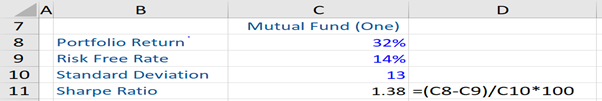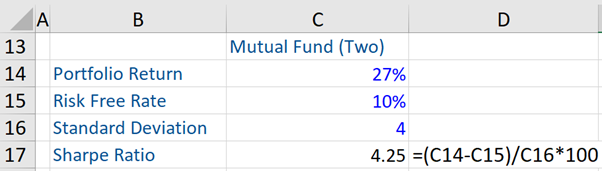## What are “Risk Adjusted Measures?”

There are four key risk adjusted performance measures – Alpha, Sharpe Ratio, Treynor Ratio, and Information Ratio. It is very important to factor in risk while evaluating a portfolio’s performance. These risk adjusted measures can help reduce the tendency of investors to simply focus on returns, without considering the investment risks.

The widely accepted principle of investment management theory and portfolio theory is that investors are risk averse. Therefore, they tend to demand additional expected returns to compensate them for any additional risks.

So, when a portfolio manager’s performance is evaluated, we want to compare returns generated by him or her with the portfolio’s corresponding risk. The aforesaid risk-adjusted measures are used for this purpose.

## Key Learning Points

• All the four risk adjusted measures reflect the investment performance of portfolio managers.
• The Treynor ratio takes into account only the systematic risk, unlike the Sharpe ratio.
• When comparing two mutual funds, the one with a higher Sharpe ratio will have performed better, relative to the risk involved in the investment.

## Types of Risk Adjusted Measures

Apha: This measures how much of the return generated by an investment portfolio is attributed or due to the portfolio manager’s investment decisions.

Having stated the above, Alpha essentially measures the excess return on a portfolio over a predetermined benchmark or market index. Investors in mutual funds or ETF’s may want a high alpha because it indicates to them that the investment decisions of the portfolio manager led to a superior return for every unit of risk taken by him or her.

In its simplest form:

Alpha  = Portfolio’s Absolute Return – Benchmark Return

As a result, if Alpha is greater (lesser) than zero, it means that the portfolio manager has outperformed (underperformed) the benchmark.

Sharpe Ratio: This is a measure used to compare the performance of mutual funds. It involves the measurement of a portfolio manager’s returns in excess of the risk-free rate (for example, the yield on US Treasury Bill) while factoring in risk taken on by the manager. The Sharpe ratio is probably the most widely used and followed risk-adjusted measure for investment funds.

The Sharpe ratio compares the excess return to the total risk, as measured by standard deviations of returns (also known as volatility) of the portfolio. This enables investors to compare investments on a risk-adjusted basis.

The formula of Sharpe ratio is:

Sharpe ratio = Rp – rf/σ

Rp – rf = Excess returns (i.e. return on a portfolio minus the risk-free rate)

σ = Standard deviation (of returns)

The higher the Sharpe ratio, the more favorable the investment return, relative to the risk being taken.

Treynor ratio: This measures a portfolio manager’s returns in excess of the risk-free rate, while also factoring in risk. This ratio compares the excess returns to systematic risk only. Therefore, the Treynor ratio is very similar to the Sharpe ratio, but instead of using the standard deviations of returns as a measure of risk, it uses beta. Beta is a measure of systematic risk and calculates the extent to which a portfolio or stock correlates or moves along with the broader market.

Treyner Ratio = Rp – rf/ β

Rp – rf = Excess returns (i.e. return on a portfolio minus the risk-free rate)

Β = Systematic risk

The higher the Treynor ratio, the better the performance of the portfolio manager.

Information ratio: This measures the excess return on a portfolio over the benchmark, which is a predetermined one, relative to the variability of that excess return. This ratio helps investors answer two questions: does the active manager outperform the passive benchmark, and is he or she able to outperform the benchmark consistently?

Information ratio = (Rp – RB)/ σ (p-B)

(Rp – RB) = Excess returns over benchmark (i.e. return on a portfolio – return on a benchmark, also called ‘’Active Return’)

σ (p-B) = Standard deviation of these excess returns – this is also known as active risk or tracking error.

The higher this ratio, the better it is considered. If the information ratio is less than zero, the active manager has failed in outperforming the benchmark.

## Sharpe Ratio – Example

Given below are two mutual funds, the first one is a Mid-Cap Stock fund and the second one is a Bluechip Fund. They have different portfolios and levels of risk. The Sharpe ratio of the first mutual fund and the second mutual fund is 1.38% and 4.25% respectively.

The second mutual fund has performed better than the first mutual fund relative to the risk involved in the investment, as it has a higher Sharpe ratio.